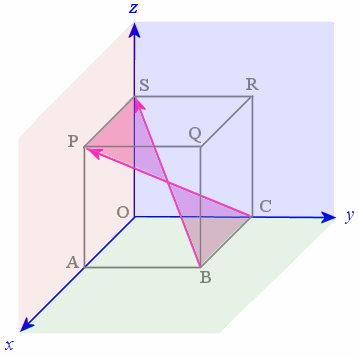Search IntMath
Close

# 8. Cross Product (aka Vector Product) of 2 Vectors

Suppose we have 2 vectors A and B. These 2 vectors lie on a plane and the unit vector n is normal (at right angles) to that plane.

The cross product (also known as the vector product) of A and B is given by:

A × B = |A| |B| sin θ n

The right hand side represents a vector at right angles to the plane containing vectors A and B.

Note: Some textbooks use the following notation for the cross product: A∧B.

### Example

In the earlier application involving a cubic box (see Vectors in 3D Application), we had a unit cube that had one corner at the origin. We found that the diagonal vectors BS and CP meet at an angle of 70.5^@ at the center of the cube.

Using the same unit cube, find the vector product of the vectors BS and CP.Using the formula

A × B = |A| |B| sin θ n

and our values from before which were

|BS| = √3
|CP| = √3
θ = 70.5°

we have:

 |BS| × |CP| = |BS| |CP| sin θ n = (√3)(√3) sin 70.5° n = 2.828 n

This means our result is a vector with magnitude 2.828 units and direction perpendicular to the plane containing BS and CP, indicated in green in the following diagram.## Problem SolverThis tool combines the power of mathematical computation engine that excels at solving mathematical formulas with the power of GPT large language models to parse and generate natural language. This creates math problem solver thats more accurate than ChatGPT, more flexible than a calculator, and faster answers than a human tutor. Learn More.